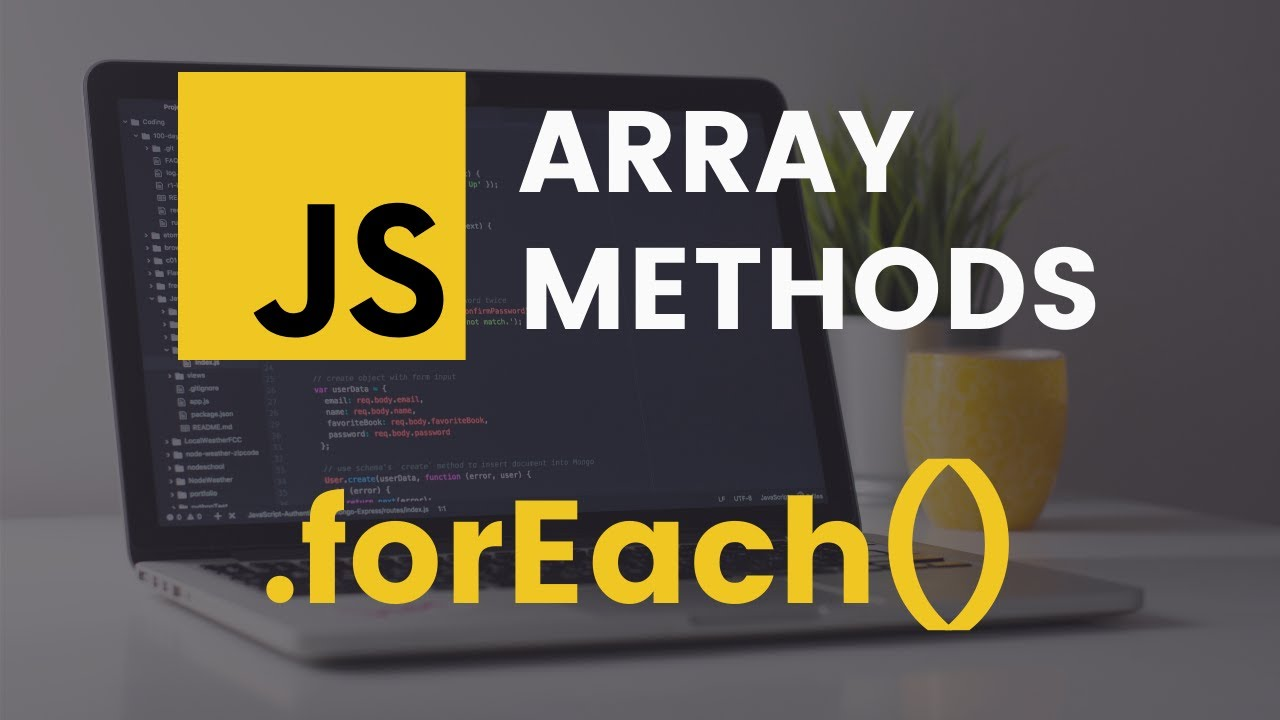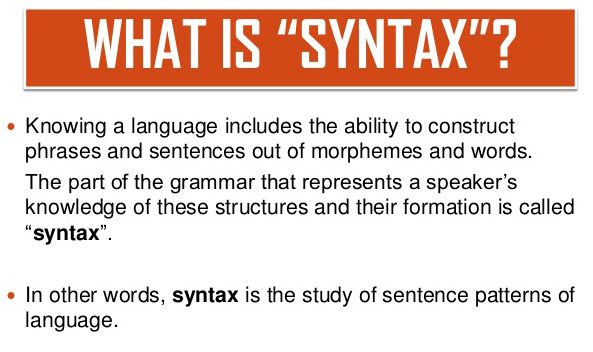Home Technology JavaScript forEach – How to Loop Through an Array in JS

# JavaScript forEach – How to Loop Through an Array in JS

34One of the various ways to loop through arrays is JavaScript forEach method. Each approach has different features and you have to determine which one to use, depending on what you do.

## What distinguishes the forEach( ) method?

The forEach method often loops through arrays, but it does so in a different way than the traditional “for loop.”

The forEach method takes the following parameters and passes a callback function for each element of an array:

Current Value (required) – The current array element’s value.

Index (optional) –The current element’s index number (optional).

Array (optional) – The array object to which the current element belongs (optional).

An example of how to use forEach() in a simple way

The following code shows how to use forEach to print all of the items in an array ()

```const arr = [1, 'two',];

arr.forEach(item => console.log(item));

// Expected output:

// 1

// two

```

For each item in the list, the forEach() method runs a function once. The function to call is passed as an argument to the process, which is called on the array object you want to control.

For each element in the array, console.log() is called in the code above.

## forEach() vs. map(), filter() and reduce()

Iteration methods in JavaScript include forEach(), map(), filter(), and reduce (). Between forEach() and the other functions, there are a few main distinctions. These methods all work in the same way, iterating through an array and applying a function to each element in the array in the order defined. The difference becomes clear when looking at the return value of these techniques. The map(), filter(), and reduce() methods all return a value, which can be either a single object or an array.

The forEach() method, on the other hand, yields an unknown result. In certain instances, such as when attempting to chain several method calls together, this can have negative consequences. As a consequence, the forEach() method is often used to serially execute a function against a set of inputs.

## SyntaxArray.forEach(callback(item, index, arr), thisValue)

The function to invoke for each object is called callback, and it has the following parameters:

• The current item is an item. The item is usually named after the type of object contained in the array to increase readability (e.g. num for numbers, car for cars etc.)
• the index is the current item’s index in the collection being processed.
• arr is the sequence that will be looped over. Since forEach() returns undefined, it’s useful if you need to make changes to the original list (the example below shows this in action)

this value helps you to modify the meaning of this object. More information can be found here.

ForEach() is used to iterate over a list in the code below.

```const words = ['hello', 'bird', 'table', 'football', 'pipe', 'code'];

const capWords = words.forEach(capitalize);

function capitalize(word, index, arr) {

arr[index] = word.toUpperCase() + word.substring(1);

}

console.log(words);

// Expected output:

// ["Hello", "Bird", "Table", "Football", "Pipe", "Code"]```

The capitalize () function is called by the forEach() method in the previous example. This function can be passed any string of terms, and it will behave similarly on the input. Until updating the contents of the original array, the method is applied to each variable in the array in turn, capitalizing each term. This makes it simple to make adjustments to an entire collection!

## When do you use forEach()?

forEach() is the easiest way to iterate over an array without breaking it and having a side effect at the same time.

Side effects include things like a mutation of an outer scope vector, I/O operations (HTTP requests), DOM manipulations, among other things.

Let’s assume we want to use forEach() to clear all input elements from the DOM.

```const inputs = document.querySelectorAll('input[type="text"]');

inputs.forEach(function(input) {

input.value = '';

});```

The callback function has the side effect of clearing the value of the input field.

It’s important to remember that you can’t normally avoid a forEach() iteration (other than a tricky way to throw an error to stop the iteration, which is a cheap hack). The process will iterate through all of the objects at all times.

The classic for or for..of is a better choice if your case necessitates an early break from the cycle.

When an array iteration produces a result with no side effects, it is preferable to use an array process like:

• map()
• reduce()
• every()
• some()

Let’s say we want to see if all of the numbers in an array are even.

The forEach() method is used in the first solution.:

let allEven = true;

```const numbers = [22, 3, 4, 10];

numbers.forEach(function(number) {

if (number % 2 === 1) {

allEven = false;

// Break here

}

});

console.log(allEven); // => false

```

If all of the numbers are even, the code decides correctly. The issue is that after finding the first odd number 3, it is impossible to crack.

For this situation, a better alternative is array.every() method:

```const numbers = [22, 3, 4, 10];

const allEven = numbers.every(function(number) {

return number % 2 === 0;

});

```
`console.log(allEven); // => false`

array.every() doesn’t only make the code shorter. It’s also the best option since the.every() method stops iterating after the first odd number is found.